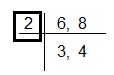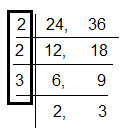QuestionAnswers

# Find HCF by finding factors: 6 and 8.A. 4B. 6C. 2D. 8Verified
128.4k+ views
Hint: A factor of a number is an exact divisor of that number.
For example: Factors of 12 = 1, 2, 3, 4, 6, 12
We can say that a number is a multiple of each of its factors
HCF is an acronym for the highest common factor.
The Highest Common Factor of two or more given numbers is the highest (or greatest) of their common factors.
It is also known as the Greatest Common Divisor (GCD).

Step 1: Find factors of 6 and 8.
Factors of 6 = 1, 2, 3.
Factors of 8 = 1, 2, 4, 8.
Step 2: Identify the common factors between 6 and 8
Common factors of 6 and 8 = 1, 2.
Step 3: Identify the highest (or greatest) factors among the common factors of 6 and 8.
A highest common factor of 6 and 8 = 2
Final answer: Thus, HCF ( 6, 8) is 2.

So, the correct answer is “Option C”.

Note: Some useful facts related to factors are as following:
1 is a factor of every number.
Every number is a factor of itself.
Every factor is less than or equal to the given number.
The number of factors of a given number are finite.
HCF can also be found using repeated division.
For example:
1) HCF of 6 and 8HCF (6, 8) = 2
2) HCF of 24 and 36HCF (24, 36) $= 2 \times 2 \times 3$ = 12